# Problem 52861. Easy Sequences 37: Natural Factorable Polynomials

A polynomial of the form: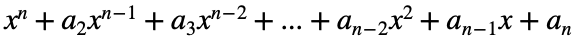, for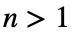, is said to be natural factorable if it can be factored into products of first degree binomials: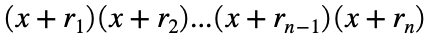, where,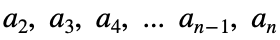and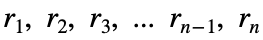are all natural numbers (i.e. integers that are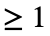).
Given an integer a, write a function that counts the number of all possible natural factorable polynomials that can be formed, wherein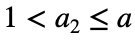.
For example, when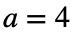, the are 7 possible natural factorable polynomials, namely: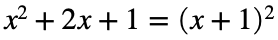;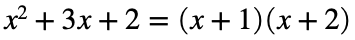;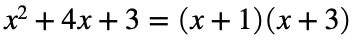;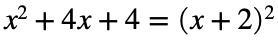;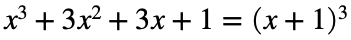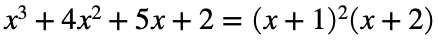; and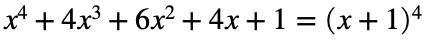Therefore the function output should be 7.

### Solution Stats

77.78% Correct | 22.22% Incorrect
Last Solution submitted on Aug 01, 2023

### Community Treasure Hunt

Find the treasures in MATLAB Central and discover how the community can help you!

Start Hunting!